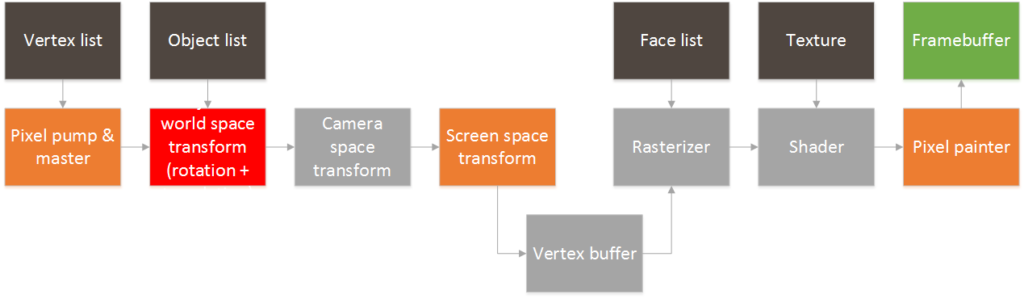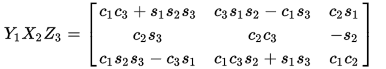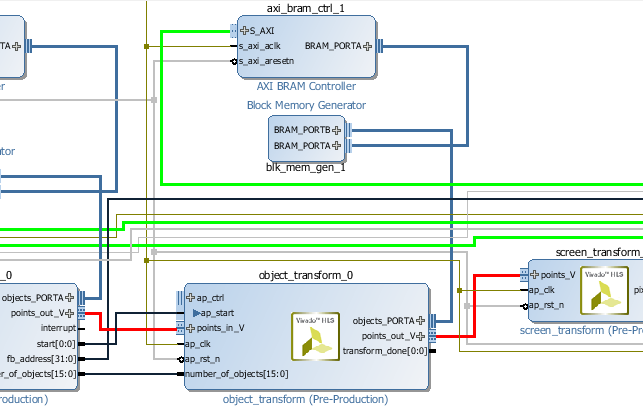# GPU Project 09 – Rotations

Sorry for the long delay between posts. Being a hobby project usually this takes low priority when other things are urgent. And actually, there have been many advances lately but I haven’t had the chance to post them. Lets go through these in order.

Although we have a fair point cloud rendering in place it really is not that useful unless we can move and rotate objects. For that purpose we have the object transform block highlighted below in red:The idea is that we can have a list of object specific parameters in a BRAM that the block will use to alter the vertex stream:

• number of vertices in the object
• position
• rotation

The block will take in vertices from the vertex pump, rotate them around the object’s axis and then add the object’s position as an offset to each vertex. The position part is actually quite simple since we only have to do an addition on each axis. The rotation part, well… no so much.

I won’t go much into detail about the deduction part of this, but in linear algebra a rotation matrix is a matrix that can be used to perform a rotation in Euclidean space. The matrix depends on the desired rotation only. In order to do the rotation, the input coordinates are multiplied by the matrix and out you get the rotated point. Here is an example for a 2d rotation matrix:3D rotation matrices can be constructed as well that depend on the three object’s angles. There are many conventions out there, but for my purposes I chose the Tait-Bryan YXZ rotation. All of the Euler rotation matrices can be easily found in the wikipedia site: https://en.wikipedia.org/wiki/Euler_angles.Where c_i represents the cosine of angle i, and s_i represents the sine of angle i. Although there are lots of multiplications here these only need to be done once per object. Once the matrix is constructed each point needs to be multiplied by the same matrix and the rotated point comes out. Given the resource limitation in the FPGA I’m using I opted to calculate the matrix in the ARM processor and do the actual matrix multiplication operations in the FPGA, since those can be parallelized and streamlined pretty well.

In HLS, I ended up splitting the block into two sections: a coefficient load section, and a matrix multiplication section. The load part is show below:

object_load: for( state = 0; state < 13; state ++){
#pragma HLS PIPELINE
switch (state){
case 0:
number_of_vertices = objects[ base_offset];
break;
case 1:
new_center.x = ((ap_fixed<32, 22, AP_TRN, AP_SAT >)objects[base_offset + 1])>>10;
break;
case 2:
new_center.y = ((ap_fixed<32, 22, AP_TRN, AP_SAT >)objects[base_offset + 2])>>10;
break;
case 3:
new_center.z = ((ap_fixed<32, 22, AP_TRN, AP_SAT >)objects[base_offset + 3])>>10;
break;

case 4:
m00 	= ((ap_fixed<32, 22, AP_TRN, AP_SAT >)objects[base_offset + 4])>>10;
break;
case 5:
m01 	= ((ap_fixed<32, 22, AP_TRN, AP_SAT >)objects[base_offset + 5])>>10;
break;
case 6:
m02 	= ((ap_fixed<32, 22, AP_TRN, AP_SAT >)objects[base_offset + 6])>>10;
break;

case 7:
m10 	= ((ap_fixed<32, 22, AP_TRN, AP_SAT >)objects[base_offset + 7])>>10;
break;
case 8:
m11 	= ((ap_fixed<32, 22, AP_TRN, AP_SAT >)objects[base_offset + 8])>>10;
break;
case 9:
m12 	= ((ap_fixed<32, 22, AP_TRN, AP_SAT >)objects[base_offset + 9])>>10;
break;

case 10:
m20 	= ((ap_fixed<32, 22, AP_TRN, AP_SAT >)objects[base_offset + 10])>>10;
break;
case 11:
m21 	= ((ap_fixed<32, 22, AP_TRN, AP_SAT >)objects[base_offset + 11])>>10;
break;
case 12:
m22 	= ((ap_fixed<32, 22, AP_TRN, AP_SAT >)objects[base_offset + 12])>>10;
break;
}
}


The matrix multiplication section is shown below. Note that I did not fully paralellize each axis given that I don’t want to burn through many DSP slices yet. I fully envision requiring a lot more for the triangle rasterization and texture shading portions.

vertex_loop: for(vertex_index = 0; vertex_index < number_of_vertices; vertex_index++ ){ //#pragma HLS PIPELINE points_in >> new_point;

filter: for( state = 0; state < 6; state ++){
linear_t tx, ty, tz;
#pragma HLS PIPELINE
switch (state){
case 0:
tx = matrix(new_point.x, new_point.y, new_point.z, m00, m01, m02);
break;
case 1:
output_point.x = tx + new_center.x;
break;
case 2:
ty = matrix(new_point.x, new_point.y, new_point.z, m10, m11, m12);
break;
case 3:
output_point.y = ty + new_center.y;
break;
case 4:
tz = matrix(new_point.x, new_point.y, new_point.z, m20, m21, m22);
break;
case 5:
output_point.z = tz + new_center.z;
break;
}
}
if (object_index == total_objects - 1) {
*transform_done = true;
}
points_out << output_point;
}


The single variable matrix multiply operation is shown below:

linear_t matrix(linear_t x, linear_t y, linear_t z, linear_t a, linear_t b, linear_t c){
return (x*a)+(y*b)+(z*c);
}


This is how it integrates into Vivado: